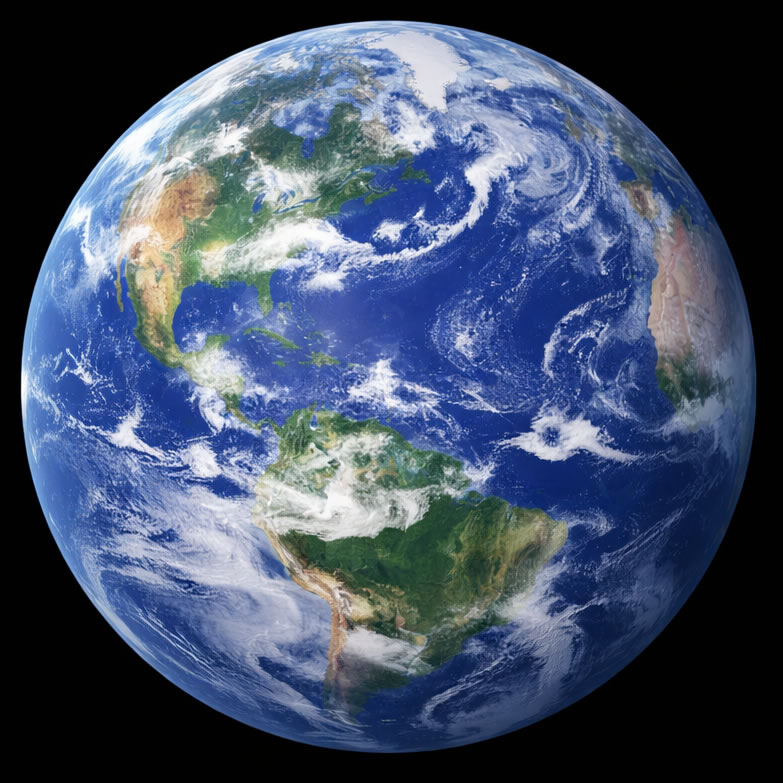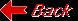Planet EarthMass (kg) 5.976e+24 Mass (Earth = 1) 1.0000e+00 Equatorial radius (km) 6,378.14 Equatorial radius (Earth = 1) 1.0000e+00 Mean density (gm/cm^3) 5.515 Mean distance from the Sun (km) 149,600,000 Mean distance from the Sun (Earth = 1) 1.0000 Rotational period (days) 0.99727 Rotational period (hours) 23.9345 Orbital period (days) 365.256 Mean orbital velocity (km/sec) 29.79 Orbital eccentricity 0.0167 Tilt of axis (degrees) 23.45 Orbital inclination (degrees) 0.000 Equatorial escape velocity (km/sec) 11.18 Equatorial surface gravity (m/sec^2) 9.78 Visual geometric albedo 0.37 Mean surface temperature 15C Atmospheric pressure (bars) 1.013 Atmospheric composition: Nitrogen 77%, Oxygen 21%, Other 2%